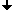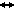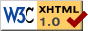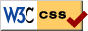Nonlinear Dynamics at the Free University Berlin

Summer 2017

# Seminar Advanced Topics in Nonlinear Dynamics

Prof. Dr. Bernold Fiedler, Hannes Stuke

## Program

 April 20, 2017 Juliana Pimentel (UFABC, Brazil) Permutation characterization for slowly non-dissipative equations. We study the longtime behavior of slowly non-dissipative scalar reaction-difusion equations. By extending known results, we are able to obtain a complete decomposition of the non compact global attractor and still manage to determine the heteroclinic connections based on the Sturm permutation method. April 27, 2017 Ismail Yenilmez (Free University Berlin) Viscid and inviscid Burger's equation. I will introduce the viscid Burger’s equation which is the inviscid Burger’s equation with a viscosity and diffusion term on the right side. I will solve the inviscid Burger’s equation by the Hopf-Cole-transformation, where the heat equation will be used. Then we look at the vanishing viscosity term, hoping to find solutions of the viscid Burger’s equation which do not blow in finite time. In the end we will recognize that the vanishing viscosity case is just a condition looking for physically best solutions. Prof. Sergey Kryzhevich (University of Nova Gorica, Slovenia) Controllability, infinite invariant measures and periodic points. We study Lipschitz maps of a non-compact metric space (in most cases, we take Euclidean spaces) with an infinite Borel invariant measure satisfying some technical conditions (e.g. Lebesgue measure in R^N). We assume that the so-called small mean drift condition is satisfied. Using techniques, developed by Burago, Ivanov and Novikov, we prove that the system is controllable, i.e. every two points can be linked by a finite chain of a delta pseudotrajectory where delta could be taken arbitrarily small. Corollaries of this result will be discussed. Particularly, we prove an analog of the famous Pugh’s closing lemma for the considered case. May 04, 2017 Prof. Pablo Mira (Universidad Politecnica de Cartagena) Proof of Alexandrov's uniqueness conjecture on prescribed curvaturespheres in R^3. In 1956 A.D. Alexandrov conjectured that if an ovaloid in R^3 satisfies an elliptic equation between its principal curvatures and ist unit normal, then any other compact surface of genus zero immersed in R^3 that satisfies the same equation is a translation of this ovaloid. Our aim in this talk is to give a proof of this conjecture. As a particular case, we also obtain an affirmative solution another classical problem: the characterization of round spheres as the unique elliptic Weingarten spheres immersed in R^3, generalizing in this way previous results by Hopf, Alexandrov, Chern or Hartman and Wintner among others. This is a joint work with Jose A. Galvez. Bernhard Brehm (Free University Berlin) tba tba May 11, 2017 Alejandro López (Free University Berlin) Connectivity properties of spindles. I want to rehearse a talk for SFB 910. It will be an overview of my current research. Nicola Vassena (Free University Berlin) Sensitivity of chemical reaction networks: an introduction. This is a rehearsal for a ignite talk I will give next week in Italy. I will try to make clear what are the main goals of the study of sensitivity of chemical reaction networks and which results have been found and which we are hoping to find. Prof. M. Scheutzow (Technical University Berlin) An introduction to stochastic delay equations. We will give a brief introduction to stochastic delay equations driven by additive or multiplicative white noise. We will present some results on the long term behavior of such equations like existence, uniqueness and asymptotic stability of invariant measures as well as exponential growth rates for certain linear equations. We will also emphasize some peculiarities of stochastic delay equations (like the lack of pathwise continuous dependence on the initial condition and the reconstruction property). We plan to make the introduction rather short and then to proceed depending on the interests of the audience. The talk will therefore be rather informal. May 18, 2017 Phillipo Lappicy Lemos Gomes (Free University) Disputation: Galois groups and fundamental groups: two sides of the same coin. Probably the most well known fact of Galois is that he died aged 20 in a duel that happened at the 31 of May of 1832. Two days before his duel, he wrote a letter explaning some of his ideas. Such ideas have flourished and bear the name of Galois theory nowadays, connecting the theory of field and its algebraic extensions to group theory. Among its most notable applications is the proof that polynomial equations of degree 5 or higher cannot be generally solvable by radicals. On the other hand, in algebraic topology, the concepts of covering spaces and fundamental groups have played a fundamental role in understanding and classifying topological spaces, ever since the Analysis situs of Poincaré in 1895. After introducing basic concepts of both these theories, we will present Grothendieck's categorical approach from 1960-61: the Galois group and the fundamental group are the same object in a higher abstract framework, and mere examples when the appropriate categories are chosen (field extensions and covering spaces, respectively). We will explore this algebraic point of view and understand its main ideas in examples. The Disputation starts 15.15. June 01, 2017 Michael Scheutzow (TU University) A brief introduction to stochastic delay equations Alejandro López (Free University Berlin) Global attractors of scalar differential delay equations. Isabelle Schneider (Free University Berlin) A mechanism of delayed feedback control. This is a SFB910 Seminar. Link to the program June 08, 2017 Adem Güngör (Free University Berlin) The analysis of a competing Two-component system. Two-component systems (TCS) in the area of molecular biology describe the response of organisms to changes in their environmental conditions. These are usually expressed by a chemical reaction network system with mass action kinetics. I analyzed a TCS which includes an additional species that acts as a competing factor. Furthermore I shortly want to mention, if similar results can be obtained at TCS with more than one additional species, which is still in progress. Yuya Tokuta (Free University Berlin) Bioconvection generated by Euglena gracilis under oscillatory illumination from the bottom. Microorganisms are known to form spatiotemporal patterns similar to those formed in the Rayleigh-Bénard model for thermal convection. Among such, Euglena gracilis form distinct patterns induced by phototaxes and sensitivity to the gradient of the light intensity. A model for the convection patterns under stationary illumination was proposed by Suematsu et al. and we modify the model to discuss patterns under oscillatory illumination. June 22, 2017 Fustel Soh Mzokou (Free University Berlin) Spatio-temporal feedback control on a two-dimensional domain. As a critical example to the spatio-temporal delayed feeback control approach for partial differential equations, it is our goal to analyse the control of the solutions' stability for the heat equation under periodic boundary conditions on a two-dimensional domain. We classify the equilibria and periodic orbits into unstable/stable and construct control terms to stabilize the unstable ones. We hope that having inserted this control term we do not change the equilibria and periodic orbits in themselves but we get all periodic orbits to become stable. Ignacio Gonzalez (Free University Berlin) Genericity and graphs. In this talk we will talk about generic subsets, its meaning and its relation with the concept of "almost everywhere". We will also work on an example of how can we obtain existence of a generic subset of vector fields attached to a coupled cell network (To do so we will use so called Sard-Smale theorems). For references read "Observation and inverse problems in coupled cell networks"- Romain Joly. Daniel Sarmiento Ferrera (Free University Berlin) Periodic travelling wave solutions for delayed Burgers equation. I will talk about the existence of stable periodic travelling wave solution for delayed Burgers via Hopf bifurcation theorem in delay equations and comment on how to determine type of bifurcation in this setting. Michael Schäfer (Free University Berlin) Guiding and measuring anisotropic diffusion. The talk is loosely related to the presentation I gave about employing anisotropic diffusion in image processing in the last semester. Introducing the structure tensor allowed us to locally distinguish between principal directions of the image, and guide the smoothing process. Similarly, real diffusion in heterogeneous media has spatially varying strength and orientation. There certainly is a correspondence between the local geometry and the diffusive tensor. Fibers restrict to one direction, and walls block a certain direction. As biological tissue like brain matter is structured on a microscopic scale, one can hope to infer this structure from obtaining the diffusive tensor of the water diffusion inside the tissue. This talk will give a short introduction to diffusion tensor imaging in brain MRI. We will focus on the problem of inferring the angle between two crossing fibers. Under the assumption of different diffusive tensors within each independent fiber and zero drift, one may decompose the water diffusion by super-position principle. As fundamental solutions of the anisotropic equations in each compartment are Gaussians, we will investigate how to infer the parameters of Gaussian mixture models by methods of Fourier and employing moments. June 29, 2017 Hannes Stuke (Free University) Disputation: Probabilistic Influence in Bayesian networks. In my talk I will give an introduction of Bayesian networks. Bayesian networks are directed acyclic graphs that represent probabilistic relationships between huge number of random variables. For example, they are applied in medicine to assist diagnosis, in biology to analyse gene expression networks, to detect production faults in manufacturing processes and in many other fields. An important property of random variables is conditional independence, i.e., can variables behave independently if we have extra knowledge about random variables elsewhere in the graph. Conditional independence not only helps to structure data, but also to derive possible graphs from a raw data set. We will show that if the set where we have this additional information separates two nodes in the graph in a certain way, then these nodes are conditionally independent. We will also discuss a converse statement which holds under generic conditions. The Disputation starts 15.15. July 06, 2017 Augusto Visintin (Trento, Italy) Mathematical models of hysteresis phenomena. (Abstract) July 13, 2017 Jia-Yuan Dai (Free University) Disputation: Generalized Latin squares. In the 18th century Choi Seok-Jeong and Euler invented Latin squares and studied their orthogonal mates, independently. Nowadays, the popular game Sudoku is a special type of Latin squares and orthogonal mates are important in modern experimental design. In April 2017, Brualdi and Dahl generalized the concepts of Latin squares and orthogonal mates by introducing alternating sign hypermatrices. In this talk we will characterize generalized Latin squares and introduce several interesting open problems. The Disputation starts 15.15. July 20, 2017 David Molle (Free University Berlin) Hale's Lyapunov function for the sunflower equation. In my talk I will present my 2nd try at proving the Lyapunov function for the sunflower equation as proposed by Hale in his book "Theory of Functional Differential Equations". Paul Dieckwisch (Free University Berlin) Long-term Behavior of Chemical Reaction Networks – The Global Attractor Conjecture. After a brief introduction into the theory of deterministically modeled chemical reaction networks we will formulate relevant questions concerning the long-term behavior of these systems. The Global Attractor Conjecture is one of the most important problems in this field and still open. We will state the conjecture and provide a coarse overview of what has already been achieved. Ismail Yenilmez (Free University Berlin) Model on currency exchange rate. In my talk I want to introduce a model with short time fluctuations of an asset, more precisely we will speak about the exchange rate. The purpose of the model is to single out possible source of those fluctuations in order to make profit for agents trading the foreign currency. Michael Schäfer (Free University Berlin) Estimating parameters of Anisotropic Diffusion. By a main result of diffusion MRI theory, the measured signal and the probability density are Gaussians, and furthermore Fourier transforms of one another. Hence, I will present a parameter estimator for Gaussian mixtures, based on Fourier methods. Abderrahim Azouani (Mohamed first University, Marocco) Feedback control and data assimilation. Since the talk of this week was about the complex Ginzburg-Landau equation, I will take this CGLE as paradigm to prove feedback control scheme for stabilizing solutions. Then, I will prove some results about our continuous data assimilation algorithm based on ideas developed for feedback controls before to talk about some work in progress related to the tumor growth using our algorithm if the time permits.

## Time and Place

Talks usually take place on Thursday at 2:15 p.m.
at the Free University Berlin
Room 140, Arnimallee 3 (rear building), 14195 Berlin.

Guests are always welcome!

## ArchiveLast change: Jul. 19, 2017
 This page strictly conforms to the XHTML1.0 standard and uses style sheets.